## Example Questions

### Example Question #5 : Complex Fractions

A cistern containinggallons of water has sprung two leaks. One leaks at a rate ofof a gallon every half hour. The second one leaks at a rate ofa gallon every fifth of an hour. In how many hours will the cistern be empty (presuming that the leaks will empty it eventually)?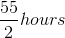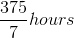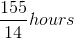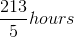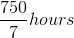Explanation:

It is best to figure out what each of the leaks are per hour. We can figure this out by adding together the two fractional rates of leaking. For the first leak, we can do this as follows: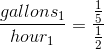This is the same as: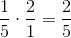For the second leak, we use the same sort of procedure: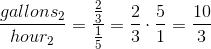Thus, our two leaks combined are: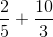The common denominator for these is; thus, we can solve: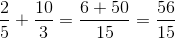Now, our equation can be set up: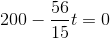, whereis the time it will take for the cistern to be emptied.

Multiply byon both sides: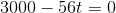Solve for: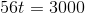Divide by: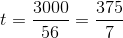### Example Question #6 : Complex Fractions

Which of the following answer choices is a value forin the following equation?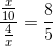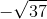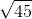Explanation:

Begin by simplifying the left side of the equation. You can do this by multiplying the numerator of the fraction by the reciprocal of its denominator: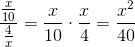Now, we know that our equation is: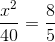Multiply both sides byand you get: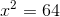Thus, by taking the square root of both sides, you get: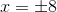Among your answers,is the only one that matches these.

### Example Question #7 : Complex Fractions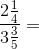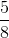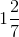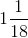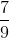Explanation:

Begin by converting both top and bottom into non-mixed fractions: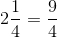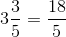So now we have: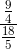In order to divide, take the fraction on the bottom, flip it, and multiply it by the fraction up top: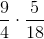Multiply straight across: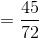Now reduce the fraction. Both top and bottom are divisible by 9 (an easy way to tell this is to see that in the original fractions we are multiplying both 9 and 18 are divisible by 9), so reduce each side by a factor of 9: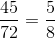The answer is.

Tired of practice problems?

Try live online GRE Math prep today.